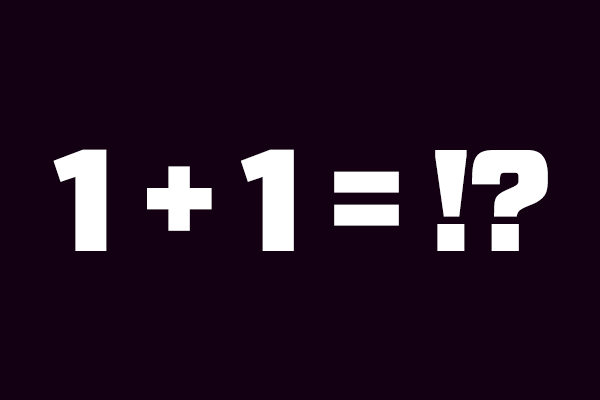Daily tips and tricks from the experts at Adafruit!If you’re porting C/C++ or Arduino code to Python or MicroPython be careful about how you deal with overflow!  In Python (and MicroPython) all numbers are arbitrarily large signed integers or floating point values.  This is great for when you just want a numeric value and don’t care about memory or performance.  A number in Python code can be any value and you never need to pick or choose how its stored like in C/C++ code.  The downside of this is that it’s tricky when you really do want a number to be constrained to a specific size or range. For example take this snippet of Arduino code and similar Python code: ```// Arduino code: uint8_t value = 250; value += 10; Serial.print("Arduino value is: "); Serial.println(value, DEC); # Python code: value = 250 value += 10 print("Python value is: {}".format(value))``` Can you guess what the output of each program would be?  The answer might surprise you: ```Arduino value is: 4 Python value is: 260``` Wow, completely different answers to the same simple math code!  What happened?  It turns out Python tried to outsmart you and its arbitrarily large integer was happy to add 10 to 250 and get the result 260.  However in the Arduino code it explicitly used a small 8-bit unsigned numeric type which can’t go beyond the value 255.  If you try to add beyond the maximum it overflows back to 0 and starts counting up again. If you deal with a lot of embedded C/C++ or Arduino code it’s very common to find algorithms and math operations that depend on this overflow behavior.  So how do you get the same behavior with Python and MicroPython code?  It turns out an easy fix is to use the bitwise AND operator (&) to extract the number of bytes you care about from a number.  For example to change the Python code above so that it behaves like the Arduino code you could write: ```# Python code with math that behaves like an 8-bit unsigned integer: value = 250 value += 10 value &= 0xFF  # This magic step uses the bitwise AND operator to extract the low 8 bits of the number. print("Python value is: {}".format(value))``` Now when you run the code you’ll get the same value as Arduino: `Python value is: 4` The &= operation converted the arbitrarily long integer in value to only the bottom 8 bits of it (i.e. what was masked by the hex value 0xFF).  This is the key step to converting a Python number to a smaller fixed-size unsigned integer.  Use this trick if you’re porting C/C++ or Arduino code to Python and see that it uses small unsigned numeric types! In other news CircuitPython had a new 0.9.0 release this week!  Check out the release page for the latest firmware images and information on all the changes.  The 0.9.0 release is a small one with minor bug fixes and polish.